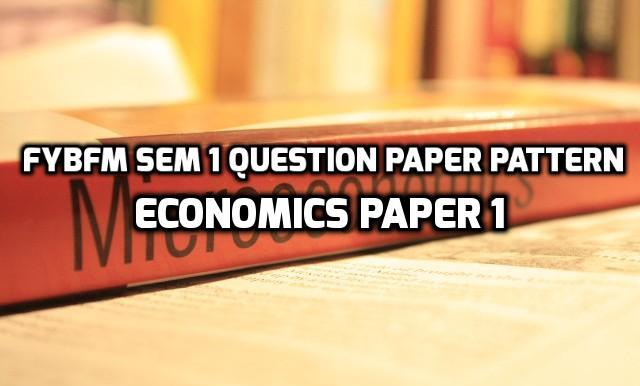# FYBFM Sem 1 Question Paper Pattern – Economics Paper 1Economics Paper 1 or Microeconomics subject includes contents and nature of economic theory, demand and supply analysis, production, cost and revenue and market analysis.

Here is the Microeconomics Question Paper Pattern:

• Maximum marks – 75
• Duration – 2.5 hours
• All questions are compulsory carrying 15 marks each.

Q.1) Objective questions: 15 marks

a) sub questions asked are 10 out of which 8 has to be answered

b) sub questions asked are 10 out of which 7 are to be answered

(the sub-questions can be multiple choice / true or false / match the columns / fill in the blanks)

Q. 2) Full length question – 15 marks (any 1 out of 2)

Q. 3) Full length question – 15 marks (any 1 out of 2)

Q. 4) Full length question – 15 marks (any 1 out of 2)

Q. 5) Full length question – 15 marks (any 1 out of 2)

The full length question of 15 marks can be 2 sub questions of 8 and 7 marks or 3 short notes of 5 marks depending upon the topics. In short notes, 3 short notes have to be answered out of 4 or 5. Options may be given within the same module so that no module is skipped by the students.